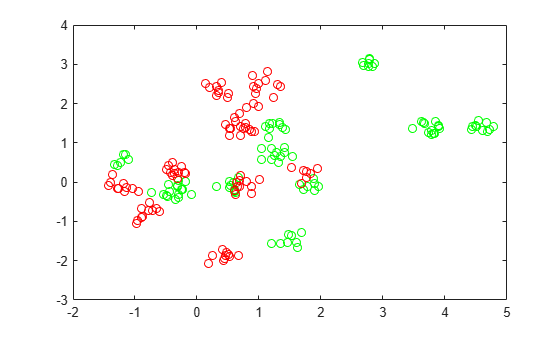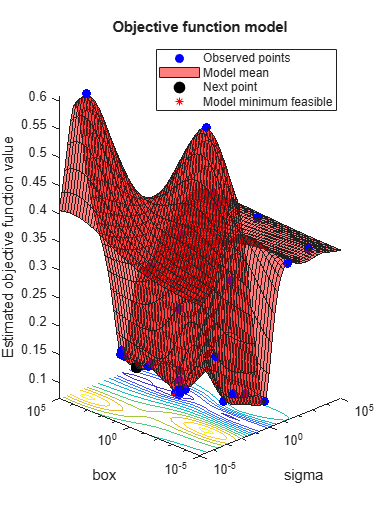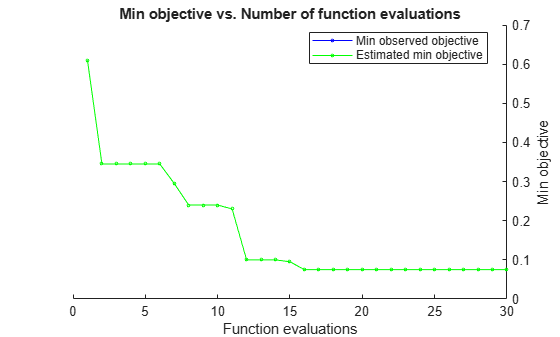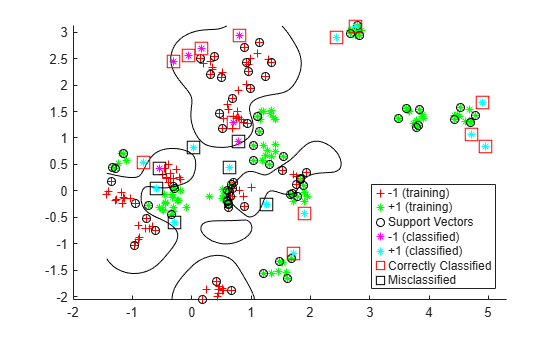# Optimize Cross-Validated Classifier Using `bayesopt`

This example shows how to optimize an SVM classification using the `bayesopt` function.

Alternatively, you can optimize a classifier by using the `OptimizeHyperparameters` name-value argument. For an example, see Optimize Classifier Fit Using Bayesian Optimization.

### Generate Data

The classification works on locations of points from a Gaussian mixture model. In The Elements of Statistical Learning, Hastie, Tibshirani, and Friedman (2009), page 17 describes the model. The model begins with generating 10 base points for a "green" class, distributed as 2-D independent normals with mean (1,0) and unit variance. It also generates 10 base points for a "red" class, distributed as 2-D independent normals with mean (0,1) and unit variance. For each class (green and red), generate 100 random points as follows:

1. Choose a base point m of the appropriate color uniformly at random.

2. Generate an independent random point with 2-D normal distribution with mean m and variance I/5, where I is the 2-by-2 identity matrix. In this example, use a variance I/50 to show the advantage of optimization more clearly.

Generate the 10 base points for each class.

```rng('default') % For reproducibility grnpop = mvnrnd([1,0],eye(2),10); redpop = mvnrnd([0,1],eye(2),10);```

View the base points.

```plot(grnpop(:,1),grnpop(:,2),'go') hold on plot(redpop(:,1),redpop(:,2),'ro') hold off```Since some red base points are close to green base points, it can be difficult to classify the data points based on location alone.

Generate the 100 data points of each class.

```redpts = zeros(100,2);grnpts = redpts; for i = 1:100 grnpts(i,:) = mvnrnd(grnpop(randi(10),:),eye(2)*0.02); redpts(i,:) = mvnrnd(redpop(randi(10),:),eye(2)*0.02); end```

View the data points.

```figure plot(grnpts(:,1),grnpts(:,2),'go') hold on plot(redpts(:,1),redpts(:,2),'ro') hold off```### Prepare Data for Classification

Put the data into one matrix, and make a vector `grp` that labels the class of each point. 1 indicates the green class, and -1 indicates the red class.

```cdata = [grnpts;redpts]; grp = ones(200,1); grp(101:200) = -1;```

### Prepare Cross-Validation

Set up a partition for cross-validation. This step fixes the train and test sets that the optimization uses at each step.

`c = cvpartition(200,'KFold',10);`

### Prepare Variables for Bayesian Optimization

Set up a function that takes an input `z = [rbf_sigma,boxconstraint]` and returns the cross-validation loss value of `z`. Take the components of `z` as positive, log-transformed variables between `1e-5` and `1e5`. Choose a wide range, because you don't know which values are likely to be good.

```sigma = optimizableVariable('sigma',[1e-5,1e5],'Transform','log'); box = optimizableVariable('box',[1e-5,1e5],'Transform','log');```

### Objective Function

This function handle computes the cross-validation loss at parameters `[sigma,box]`. For details, see `kfoldLoss`.

`bayesopt` passes the variable `z` to the objective function as a one-row table.

```minfn = @(z)kfoldLoss(fitcsvm(cdata,grp,'CVPartition',c,... 'KernelFunction','rbf','BoxConstraint',z.box,... 'KernelScale',z.sigma));```

### Optimize Classifier

Search for the best parameters `[sigma,box]` using `bayesopt`. For reproducibility, choose the `'expected-improvement-plus'` acquisition function. The default acquisition function depends on run time, and so can give varying results.

```results = bayesopt(minfn,[sigma,box],'IsObjectiveDeterministic',true,... 'AcquisitionFunctionName','expected-improvement-plus')```
```|=====================================================================================================| | Iter | Eval | Objective | Objective | BestSoFar | BestSoFar | sigma | box | | | result | | runtime | (observed) | (estim.) | | | |=====================================================================================================| | 1 | Best | 0.61 | 0.16234 | 0.61 | 0.61 | 0.00013375 | 13929 | | 2 | Best | 0.345 | 0.19695 | 0.345 | 0.345 | 24526 | 1.936 | | 3 | Accept | 0.61 | 0.47093 | 0.345 | 0.345 | 0.0026459 | 0.00084929 | | 4 | Accept | 0.345 | 0.23638 | 0.345 | 0.345 | 3506.3 | 6.7427e-05 | | 5 | Accept | 0.345 | 0.24992 | 0.345 | 0.345 | 9135.2 | 571.87 | | 6 | Accept | 0.345 | 0.23028 | 0.345 | 0.345 | 99701 | 10223 | | 7 | Best | 0.295 | 0.37581 | 0.295 | 0.295 | 455.88 | 9957.4 | | 8 | Best | 0.24 | 1.494 | 0.24 | 0.24 | 31.56 | 99389 | | 9 | Accept | 0.24 | 1.9341 | 0.24 | 0.24 | 10.451 | 64429 | | 10 | Accept | 0.35 | 0.28809 | 0.24 | 0.24 | 17.331 | 1.0264e-05 | | 11 | Best | 0.23 | 1.2079 | 0.23 | 0.23 | 16.005 | 90155 | | 12 | Best | 0.1 | 0.26949 | 0.1 | 0.1 | 0.36562 | 80878 | | 13 | Accept | 0.115 | 0.3364 | 0.1 | 0.1 | 0.1793 | 68459 | | 14 | Accept | 0.105 | 0.47601 | 0.1 | 0.1 | 0.2267 | 95421 | | 15 | Best | 0.095 | 0.22541 | 0.095 | 0.095 | 0.28999 | 0.0058227 | | 16 | Best | 0.075 | 0.51288 | 0.075 | 0.075 | 0.30554 | 8.9017 | | 17 | Accept | 0.085 | 0.21812 | 0.075 | 0.075 | 0.41122 | 4.4476 | | 18 | Accept | 0.085 | 0.20661 | 0.075 | 0.075 | 0.25565 | 7.8038 | | 19 | Accept | 0.075 | 0.31366 | 0.075 | 0.075 | 0.32869 | 18.076 | | 20 | Accept | 0.085 | 0.19086 | 0.075 | 0.075 | 0.32442 | 5.2118 | |=====================================================================================================| | Iter | Eval | Objective | Objective | BestSoFar | BestSoFar | sigma | box | | | result | | runtime | (observed) | (estim.) | | | |=====================================================================================================| | 21 | Accept | 0.3 | 0.23116 | 0.075 | 0.075 | 1.3592 | 0.0098067 | | 22 | Accept | 0.12 | 0.25542 | 0.075 | 0.075 | 0.17515 | 0.00070913 | | 23 | Accept | 0.175 | 0.46643 | 0.075 | 0.075 | 0.1252 | 0.010749 | | 24 | Accept | 0.105 | 0.48899 | 0.075 | 0.075 | 1.1664 | 31.13 | | 25 | Accept | 0.1 | 0.3266 | 0.075 | 0.075 | 0.57465 | 2013.8 | | 26 | Accept | 0.12 | 0.2722 | 0.075 | 0.075 | 0.42922 | 1.1602e-05 | | 27 | Accept | 0.12 | 0.28654 | 0.075 | 0.075 | 0.42956 | 0.00027218 | | 28 | Accept | 0.095 | 0.47424 | 0.075 | 0.075 | 0.4806 | 13.452 | | 29 | Accept | 0.105 | 0.33277 | 0.075 | 0.075 | 0.19755 | 943.87 | | 30 | Accept | 0.205 | 0.2595 | 0.075 | 0.075 | 3.5051 | 93.492 | __________________________________________________________ Optimization completed. MaxObjectiveEvaluations of 30 reached. Total function evaluations: 30 Total elapsed time: 49.9565 seconds Total objective function evaluation time: 12.99 Best observed feasible point: sigma box _______ ______ 0.30554 8.9017 Observed objective function value = 0.075 Estimated objective function value = 0.075 Function evaluation time = 0.51288 Best estimated feasible point (according to models): sigma box _______ ______ 0.32869 18.076 Estimated objective function value = 0.075 Estimated function evaluation time = 0.30586 ``````results = BayesianOptimization with properties: ObjectiveFcn: @(z)kfoldLoss(fitcsvm(cdata,grp,'CVPartition',c,'KernelFunction','rbf','BoxConstraint',z.box,'KernelScale',z.sigma)) VariableDescriptions: [1x2 optimizableVariable] Options: [1x1 struct] MinObjective: 0.0750 XAtMinObjective: [1x2 table] MinEstimatedObjective: 0.0750 XAtMinEstimatedObjective: [1x2 table] NumObjectiveEvaluations: 30 TotalElapsedTime: 49.9565 NextPoint: [1x2 table] XTrace: [30x2 table] ObjectiveTrace: [30x1 double] ConstraintsTrace: [] UserDataTrace: {30x1 cell} ObjectiveEvaluationTimeTrace: [30x1 double] IterationTimeTrace: [30x1 double] ErrorTrace: [30x1 double] FeasibilityTrace: [30x1 logical] FeasibilityProbabilityTrace: [30x1 double] IndexOfMinimumTrace: [30x1 double] ObjectiveMinimumTrace: [30x1 double] EstimatedObjectiveMinimumTrace: [30x1 double] ```

Obtain the best estimated feasible point from the `XAtMinEstimatedObjective` property or by using the `bestPoint` function. By default, the `bestPoint` function uses the `'min-visited-upper-confidence-interval'` criterion. For details, see the Criterion name-value argument of `bestPoint`.

`results.XAtMinEstimatedObjective`
```ans=1×2 table sigma box _______ ______ 0.32869 18.076 ```
`z = bestPoint(results)`
```z=1×2 table sigma box _______ ______ 0.32869 18.076 ```

Use the best point to train a new, optimized SVM classifier.

```SVMModel = fitcsvm(cdata,grp,'KernelFunction','rbf', ... 'KernelScale',z.sigma,'BoxConstraint',z.box);```

To visualize the support vector classifier, predict scores over a grid.

```d = 0.02; [x1Grid,x2Grid] = meshgrid(min(cdata(:,1)):d:max(cdata(:,1)), ... min(cdata(:,2)):d:max(cdata(:,2))); xGrid = [x1Grid(:),x2Grid(:)]; [~,scores] = predict(SVMModel,xGrid);```

Plot the classification boundaries.

```figure h(1:2) = gscatter(cdata(:,1),cdata(:,2),grp,'rg','+*'); hold on h(3) = plot(cdata(SVMModel.IsSupportVector,1) ,... cdata(SVMModel.IsSupportVector,2),'ko'); contour(x1Grid,x2Grid,reshape(scores(:,2),size(x1Grid)),[0 0],'k'); legend(h,{'-1','+1','Support Vectors'},'Location','Southeast');```### Evaluate Accuracy on New Data

Generate and classify new test data points.

```grnobj = gmdistribution(grnpop,.2*eye(2)); redobj = gmdistribution(redpop,.2*eye(2)); newData = random(grnobj,10); newData = [newData;random(redobj,10)]; grpData = ones(20,1); % green = 1 grpData(11:20) = -1; % red = -1 v = predict(SVMModel,newData);```

Compute the misclassification rates on the test data set.

`L = loss(SVMModel,newData,grpData)`
```L = 0.3500 ```

See which new data points are correctly classified. Circle the correctly classified points in red, and the incorrectly classified points in black.

```h(4:5) = gscatter(newData(:,1),newData(:,2),v,'mc','**'); mydiff = (v == grpData); % Classified correctly for ii = mydiff % Plot red squares around correct pts h(6) = plot(newData(ii,1),newData(ii,2),'rs','MarkerSize',12); end for ii = not(mydiff) % Plot black squares around incorrect pts h(7) = plot(newData(ii,1),newData(ii,2),'ks','MarkerSize',12); end legend(h,{'-1 (training)','+1 (training)','Support Vectors', ... '-1 (classified)','+1 (classified)', ... 'Correctly Classified','Misclassified'}, ... 'Location','Southeast'); hold off```